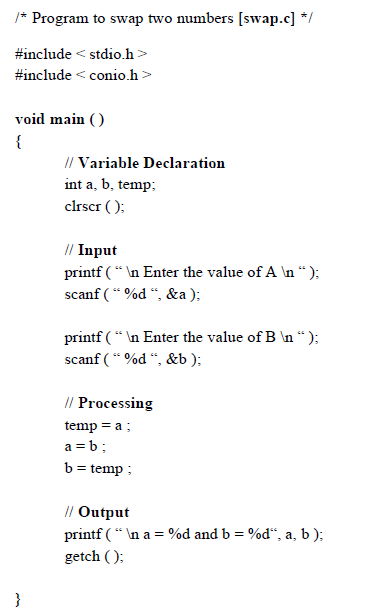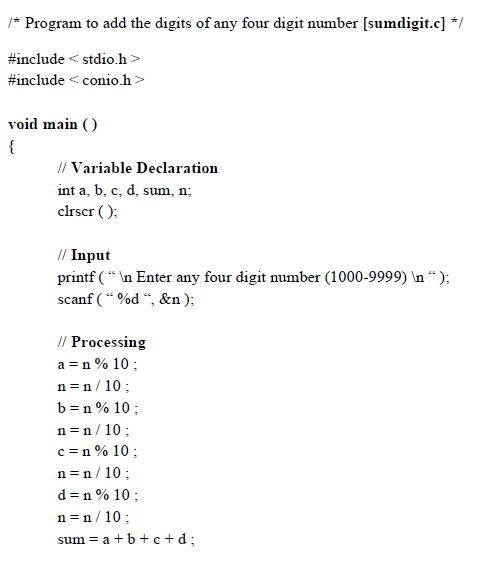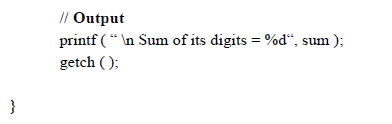## More C programs

View

Two numbers are input through the keyboard. Write algorithm, draw flowchart and write C program to swap both numbers.Output-

Enter the value of A  10
Enter the value of B  20
a = 20

b = 10

A four digit number ‘n’ is input through the keyboard. Write algorithm, draw flowchart and write C program to display the sum of its digits.

[ Hint – Let  n = 3457 then sum of its digits = 3 + 4 + 5 + 7 = 19 ]Output

Enter any four digit number (1000-9999)  9875
Sum of its digits = 29